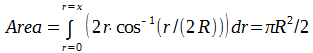# Cow Grazing in a Circular Pasture Problem: Solution by Calculus

```"A cow is attached by a rope to a point on the perimeter
of a circular field. How long should the rope be in terms
of the radius of the field so that the cow can graze in
exactly half of the field?"

This problem has been around in one form or another
for a long time.  You can find many solutions to it
on on the internet.  But all of the solutions I've seen
are geometric solutions, using plane geometry.  They
all chop up the pasture into various shapes and add up
the area of each one.

I have found a solution using calculus that I think is
a lot simpler and more elegant.  This solution defines
a differential of area that is centered around the point
on the perimeter of the pasture where one end of the
rope is tied.  Then it integrates from the origin (zero)
until the rope is long enough to allow the cow to graze
on half the area of the circular pasture.
Here's the derivation:

Let R be the radius of the cow pasture.
Let r be the length of the rope.
Let Θ be the angle between a line from the point where
the rope is tied on the perimeter of the pasture to the
center of the pasture, and a line of length r from where
the rope is tied on the perimeter to the edge of the
pasture.  See diagram: ``````1. BC is a circular arc of radius r centered at point A.
The length L of BC is 2Θr.
2. Cos(Θ) = AB/AD = r/(2R), so Θ = cos-1(r/(2R)).
(Note: Angle ABD is a right angle because it intercepts
a 180 degree arc.)
3. So L = 2Θr = 2r * cos-1(r/(2R)).
4. Consequently, one form of a differential of area of
the pasture is dArea = 2r * cos-1(r/(2R))dr.

We want to integrate this differential along AD starting
at point A until r is long enough so that half the area
of the pasture is enclosed, so we need to solve for x:Wolfram’s online integrator, integrals.wolfram.com, easily solves
the integral portion of this equation if you substitute K for R
in the integral.  Then you can let R = 1 and use WolframAlpha to
solve for the ratio of r to R that will result in half of the
area of the pasture.  The answer, to 4 decimal places is
r = R * 1.1587
```